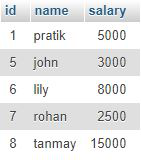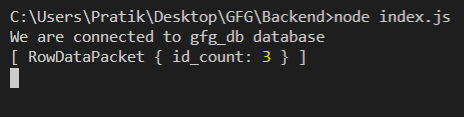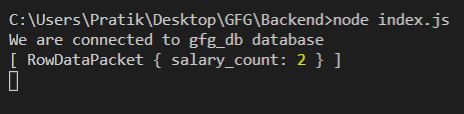Skip to content
Related Articles
NodeJS MySQL Count() Function
• Last Updated : 17 Feb, 2021

We use the COUNT() function in MySQL to count a number of rows in a column satisfying the WHERE clause.

Syntax:

`COUNT([column_name])`

Parameters: COUNT() function accepts a single parameter as mentioned above and described below.

• column_name: Column’s name from which we have to return the count value.

Module Installation: Install the mysql module using the following command:

`npm install mysql`

Database: Our SQL publishers table preview with sample data is shown below:Example 1: Count publishers having id greater than 5

## index.js

 `const mysql = require(``"mysql"``);`` ` `let db_con  = mysql.createConnection({``    ``host: ``"localhost"``,``    ``user: ``"root"``,``    ``password: ``''``,``    ``database: ``'gfg_db'``});`` ` `db_con.connect((err) => {``    ``if` `(err) {``      ``console.log(``"Database Connection Failed !!!"``, err);``      ``return``;``    ``}`` ` `    ``console.log(``"We are connected to gfg_db database"``);`` ` `    ``// Here is the query``    ``let query = ``"SELECT COUNT(id) AS id_count FROM publishers WHERE id > 5"``;`` ` `    ``db_con.query(query, (err, rows) => {``        ``if``(err) ``throw` `err;`` ` `        ``console.log(rows);``    ``});``});`

Run the index.js file using the following command:

`node index.js`

Output:Example 2:

## index.js

 `const mysql = require(``"mysql"``);`` ` `let db_con  = mysql.createConnection({``    ``host: ``"localhost"``,``    ``user: ``"root"``,``    ``password: ``''``,``    ``database: ``'gfg_db'``});`` ` `db_con.connect((err) => {``    ``if` `(err) {``      ``console.log(``"Database Connection Failed !!!"``, err);``      ``return``;``    ``}`` ` `    ``console.log(``"We are connected to gfg_db database"``);`` ` `    ``// Here is the query``    ``let query = ``"SELECT COUNT(salary) AS salary_count FROM ``                 ``publishers WHERE salary BETWEEN 5000 AND 10000"``;`` ` `    ``db_con.query(query, (err, rows) => {``        ``if``(err) ``throw` `err;`` ` `        ``console.log(rows);``    ``});``});`

Run the index.js file using the following command:

`node index.js`

Output:My Personal Notes arrow_drop_up